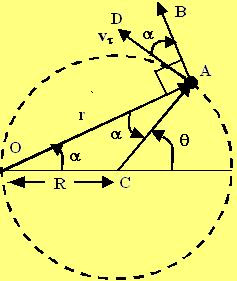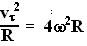## Tuesday, July 24, 2007

### Irodov Problem 1.43For solving this problem we will rely heavily on the figure provided. The particle moves along the circle shown in the figure and its angular velocity relative to point O is constant. Letbe the instantaneous tangential velocity of the particle. The component of tangential velocity in a direction perpendicular to the vector OA (along AB) is given by,. Thus, from the basic definition of angular velocity of a particle relative to a point, the angular velocity of the particle relative to O is given by,.

Form basic geometry in the figure we have,In other words,Now the particle moves along the circle thus, there is no component of the velocity normal to its direction of motion, ie Vn = 0. In other words, the velocity of the particle is same as the tangential component. Since there is no tangential acceleration for the particle (since it moves at a constant tangential velocity), the only acceleration experienced by the body is the centripetal acceleration given by,